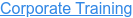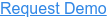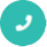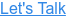#### 15+ Categories. 500+ IT Courses to Learn.# Machine Learning Interview Questions and Answers

by Mohammed, on May 18, 2018 2:17:24 PM## Q1. What is Machine learning?

Ans: Machine learning is a field of computer science that deals with system programming to learn and improve with experience.
For example: Robots are coded so that they can perform the task based on data they collect from sensors. It robotically learns programs from data

## Q2. What is the Box-Cox transformation used for?

Ans: The Box-Cox transformation is a generalized "power transformation" that transforms data to make the distribution more normal.
For example, when its lambda parameter is 0, it's equivalent to the log-transformation.
It's used to stabilize the variance (eliminate heteroskedasticity) and normalize the distribution.

## Q3. What is ‘Overfitting’ in Machine learning?

Ans: In machine learning, when a statistical model defines random error of underlying relationship ‘overfitting’ occurs.  When a model is exceptionally complex, overfitting is generally observed, because of having too many factors with respect to the number of training data types. The model shows poor performance which has been overfit.

## Q4. What are the different Algorithm techniques in Machine Learning?

Ans: The different types of techniques in Machine Learning are:

• Supervised Learning
• Semi-supervised Learning
• Unsupervised Learning
• Transduction
• Reinforcement Learning

## Q5. How is KNN different from k-means clustering?

Ans: K-Nearest Neighbors is a supervised classification algorithm, while k-means clustering is an unsupervised clustering algorithm. While the mechanisms may seem similar at first, what this really means is that in order for K-Nearest Neighbors to work, you need labeled data you want to classify an unlabeled point into (thus the nearest neighbor part). K-means clustering requires only a set of unlabeled points and a threshold: the algorithm will take unlabeled points and gradually learn how to cluster them into groups by computing the mean of the distance between different points.
The critical difference here is that KNN needs labeled points and is thus supervised learning, while k-means doesn’t — and is thus unsupervised learning.

## Q6. Mention the difference between Data Mining and Machine learning?

Ans:

Data mining: It is defined as the process in which the unstructured data tries to abstract knowledge or unknown interesting patterns.  During this machine process, learning algorithms are used.
Machine learning: It relates with the study, design and development of the algorithms that give processors the ability to learn without being openly programmed.

## Q7. What are the five popular algorithms of Machine Learning?

Ans: Five popular algorithms are:

1. Decision Trees
2. Probabilistic networks
3. Neural Networks (back propagation)
4. Support vector machines
5. Nearest Neighbor

## Q8. Define precision and recall.

Ans: Recall is also known as the true positive rate: the amount of positives your model claims compared to the actual number of positives there are throughout the data. Precision is also known as the positive predictive value, and it is a measure of the amount of accurate positives your model claims compared to the number of positives it actually claims. It can be easier to think of recall and precision in the context of a case where you’ve predicted that there were 10 apples and 5 oranges in a case of 10 apples. You’d have perfect recall (there are actually 10 apples, and you predicted there would be 10) but 66.7% precision because out of the 15 events you predicted, only 10 (the apples) are correct.

## Q9. Why is “Naive” Bayes naive?

Ans: Despite its practical applications, especially in text mining, Naive Bayes is considered “Naive” because it makes an assumption that is virtually impossible to see in real-life data: the conditional probability is calculated as the pure product of the individual probabilities of components. This implies the absolute independence of features — a condition probably never met in real life.
As a Quora commenter put it whimsically, a Naive Bayes classifier that figured out that you liked pickles and ice cream would probably naively recommend you a pickle ice cream.

## Q10. Why overfitting happens?

Ans: The possibility of overfitting happens as the criteria used for training the model is not the same as the criteria used to judge the efficiency of a model.

## Q11. What is inductive machine learning?

Ans: The inductive machine learning implicates the process of learning by examples, where a system, from a set of observed instances tries to induce a general rule.

## Q12. What is the standard approach to supervised learning?

Ans: Split the set of example into the training set and the test is the standard approach to supervised learning is.## Q13. What’s your favorite algorithm, and can you explain it to me in less than a minute?

Ans: This type of question tests your understanding of how to communicate complex and technical nuances with poise and the ability to summarize quickly and efficiently. Make sure you have a choice and make sure you can explain different algorithms so simply and effectively that a five-year-old could grasp the basics!

## Q14. What’s the difference between Type I and Type II error?

Ans: Don’t think that this is a trick question! Many machine learning interview questions will be an attempt to lob basic questions at you just to make sure you’re on top of your game and you’ve prepared all of your bases.
Type I error is a false positive, while Type II error is a false negative. Briefly stated, Type I error means claiming something has happened when it hasn’t, while Type II error means that you claim nothing is happening when in fact something is.
A clever way to think about this is to think of Type I error as telling a man he is pregnant, while Type II error means you tell a pregnant woman she isn’t carrying a baby.

## Q15. In what areas Pattern Recognition is used?

Ans: Pattern Recognition can be used in the following areas:

• Computer Vision
• Data Mining
• Speech Recognition
• Informal Retrieval
• Statistics
• Bio-Informatics## Q16. What’s a Fourier transform?

Ans: A Fourier transform is a generic method to decompose generic functions into a superposition of symmetric functions. Or as this more intuitive tutorial puts it, given a smoothie, it’s how we find the recipe. The Fourier transform finds the set of cycle speeds, amplitudes and phases to match any time signal. A Fourier transform converts a signal from time to frequency domain — it’s a very common way to extract features from audio signals or other time series such as sensor data.

## Q17. How can you avoid overfitting?

Ans: By using a lot of data overfitting can be avoided, overfitting happens relatively as you have a small dataset, and you try to learn from it. But if you have a small database and you are forced to come with a model based on that. In such situation, you can use a technique known as cross validation. In this method the dataset splits into two section, testing and training datasets, the testing dataset will only test the model while, in training dataset, the data points will come up with the model.
In this technique, a model is usually given a dataset of a known data on which training (training data set) is run and a dataset of unknown data against which the model is tested. The idea of cross validation is to define a dataset to “test” the model in the training phase.

## Q18. What are the three stages to build the hypotheses or model in machine learning?

Ans:

• Model building
• Applying the model
• Model testing.

## Q19. What’s the difference between a generative and discriminative model?

Ans: A generative model will learn categories of data while a discriminative model will simply learn the distinction between different categories of data. Discriminative models will generally outperform generative models on classification tasks.

## Q20. How is a decision tree pruned?

Ans: Pruning is what happens in decision trees when branches that have weak predictive power are removed in order to reduce the complexity of the model and increase the predictive accuracy of a decision tree model. Pruning can happen bottom-up and top-down, with approaches such as reduced error pruning and cost complexity pruning.
Reduced error pruning is perhaps the simplest version: replace each node. If it doesn’t decrease predictive accuracy, keep it pruned. While simple, this heuristic actually comes pretty close to an approach that would optimize for maximum accuracy.

## Q21. How would you handle an imbalanced dataset?

Ans: An imbalanced dataset is when you have, for example, a classification test and 90% of the data is in one class. That leads to problems: an accuracy of 90% can be skewed if you have no predictive power on the other category of data! Here are a few tactics to get over the hump:
1. Collect more data to even the imbalances in the dataset.
2. Resample the dataset to correct for imbalances.
3. Try a different algorithm altogether on your dataset.

What’s important here is that you have a keen sense for what damage an unbalanced dataset can cause, and how to balance that.

Related Interview Questions...